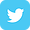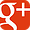708.453.2570

Request a Quote »

# How to Measure Extension Spring Tension

## What Is Extension Spring Initial Tension?

Initial tension is the tension wound between the coils of an extension spring. It keeps the coils closed and requires outside force before the coils open. To release initial tension, you must extend an extension spring until light shines through the coils.

Unlike a compression spring, an extension spring can have a preload at zero deflection. The amount of force an extension spring produces is an important part of its design and production, as it affects spring performance. Find out how to measure a spring’s initial tension below.

## Initial Tension Formula: How to Measure Spring Tension

You can calculate initial tension of an extension spring with a simple formula:

P1 = P – R(T)

The variables in this equation represent the following:

• P1 = Initial Tension
• R = Rate
• T = Travel

For example, where R=10 pounds per inch, P=20 pounds and T=1.5 inches, you can find the pounds of force for your spring’s initial tension. Simply multiply 10 by 1.5 and subtract from 20 to get 5 pounds of force (5lbf).

P1 = 20 – 10 * 1.5

P1 = 20 – 10

P1 = 5lbf

To understand more about this equation and extension spring terminology, explore our glossary of spring and wire form terms.

If you need assistance calculating initial tension or learning how to measure a spring, contact us. At Master Spring, we help OEMs determine the best spring or wire form for their needs. To get started, request a free extension spring quote today, and include the following information:

• Heights or lengths in the spring’s free position, installed position and fully-loaded position
• Dimension of the cavity (hole) that the spring fits into
• Dimension of the rod or mandrel the spring fits over
• Description of the mating part© Master Spring and Wire Form Co. 1340 Ardmore Ave., Itasca, IL 60143 Phone: 708.453.2570 | Fax: 708-453-6420